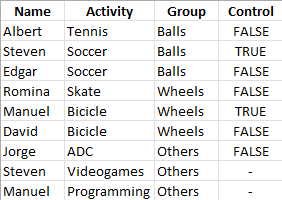cancel
Showing results for
Did you mean:

Fabric is Generally Available. Browse Fabric Presentations. Work towards your Fabric certification with the Cloud Skills Challenge.Helper I

## Mesure to count repetitions

Hi Community!

I have been practicing and feel stucked in this part, if someone could give me some guide i will be grateful.

I have the next example table:I would like to transfer this logic to Power BI. I have a column name, an activity and a group. I want to know if a Person has "any group" and also the group "Others", for example if the person is on Wheels and Balls group it counts as False, is True just when is in any group and also in Others.

My mind is blowing, i will appreciate any help!

1 ACCEPTED SOLUTIONHelper I

Thanks for the help!!

At the beggining it doesnt work because i think it was taking the last record boolean, so i add this line and it works.

ALLEXCEPT('Table','Table'[Name])

So the code finally is this way:

Control =
VAR other = CALCULATE(COUNTROWS('Table');
'Table'[Name] = EARLIER('Table'[Name]) &&
'Table'[Group] = "Others", ALLEXCEPT('Table','Table'[Name])) > 0
VAR some_group = CALCULATE(COUNTROWS('Table');
'Table'[Name] = EARLIER('Table'[Name]) &&
'Table'[Group] <> "Others", ALLEXCEPT('Table','Table'[Name])) > 0
RETURN
AND(other;some_group)

3 REPLIES 3Super User

Hi,

Try these measures

``Count of activites and group = CALCULATE(COUNTROWS(Data),ALLEXCEPT(Data,Data[name]))``
``Count of Other group = CALCULATE(COUNTROWS(Data),ALL(Data[Activity]),Data[Group]="Others")``
``Control = if(max(Data[Group])="Others","-",[Count of activites and group]<>1)``

Hope this helps.Regards,
Ashish Mathur
http://www.ashishmathur.comFrequent Visitor

Hi !

I think you can do this as a new column

Control =
VAR other = CALCULATE(COUNTROWS('Table');
'Table'[Name] = EARLIER('Table'[Name]) &&
'Table'[Group] = "Others") > 0
VAR some_group = CALCULATE(COUNTROWS('Table');
'Table'[Name] = EARLIER('Table'[Name]) &&
'Table'[Group] <> "Others") > 0
RETURN
AND(other;some_group)

Cheers
PAGHelper I

Thanks for the help!!

At the beggining it doesnt work because i think it was taking the last record boolean, so i add this line and it works.

ALLEXCEPT('Table','Table'[Name])

So the code finally is this way:

Control =
VAR other = CALCULATE(COUNTROWS('Table');
'Table'[Name] = EARLIER('Table'[Name]) &&
'Table'[Group] = "Others", ALLEXCEPT('Table','Table'[Name])) > 0
VAR some_group = CALCULATE(COUNTROWS('Table');
'Table'[Name] = EARLIER('Table'[Name]) &&
'Table'[Group] <> "Others", ALLEXCEPT('Table','Table'[Name])) > 0
RETURN
AND(other;some_group)Announcements#### Power BI Monthly Update - November 2023

Check out the November 2023 Power BI update to learn about new features.#### The largest Power BI and Fabric virtual conference

130+ sessions, 130+ speakers, Product managers, MVPs, and experts. All about Power BI and Fabric. Attend online or watch the recordings.Top Solution Authors
Top Kudoed Authors
Users online (1,938)